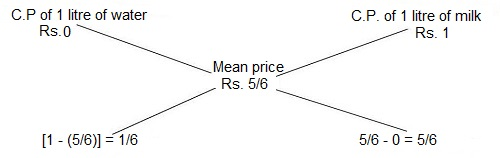Alligation and Mixture Solved Problems - ObjectiveBooks

# Alligation and Mixture Solved Problems

Question No. 01
The milk and water in two vessels A and B are in the ratio 4: 3 and 2: 3 respectively. In what ratio, the liquids in both the vessels be mixed to obtain a new mixture in vessel C containing half milk and half water?

Solution:
Let the C.P. of milk be Rs. 1 per litre
Milk in 1 litre mixture of A = 4/7 litre; Milk in 1 litre mixture of B = 2/5 litre;
Milk in 1 litre mixture of C = ½ litre
C.P. of 1 litre mixture in A = Rs. 4/7
C.P. of 1 litre mixture in B = Rs. 2/5
Mean price = Rs. 1/2

By the rule of alligation, we have:Alligation and Mixture Solution-01
Therefore, required ratio = (1/10): (1/14) = 7: 5

Question No. 02
In what ratio must water be mixed with milk to gain 20 % by selling the mixture at cost price?

Solution:
Let C.P. of milk be Rs. 1 per litre.
Then, S.P. of 1 litre of mixture = Rs. 1.
Gain obtained = 20%.
Therefore, C.P. of 1 litre of mixture = Rs. [(100/120) × 1] = 5/6

By the rule of alligation, we have:Alligation and Mixture Solution-02
Therefore, Ratio of water and milk = (1/6): (5/6) = 1: 5.

Question No. 03
In what ratio must rice at Rs. 9.30 per kg be mixed with rice at Rs. 10.80 per kg so that the mixture be worth Rs. 10 per kg?

Solution: By the rule of alligation, we have:Alligation and Mixture Solution-03
(Cheaper quantity): (Dearer quantity) = (d - m): (m - c).
=> (1080 - 1000):  (1000 - 930) => 80: 70 =>8: 7
Therefore, required ratio =8: 7.

Question No. 04
A vessel is filled with liquid, 3 parts of which are water and 5 parts acid. How much of the mixture must be drawn off and replaced with water so that the mixture may be half water and half acid?

Solution:
Suppose the vessel initially contains 8 litres of liquid.
Let, ‘x’ litres of this liquid be replaced with water.
Quantity of water in new mixture = [3 - (3x/8) + x] litres
Quantity of acid in new mixture = [5 - (5x/8)] litres
Therefore, [3 - (3x/8) + x] = [5 - (5x/8)]
=> 5x + 24 = 40 - 5x => 10x = 16 => x = 8/5
So, parts of mixture replaced = (8/5) × (1/8) = 1/5.

Question No. 05
Coffee wroth Rs. 126 per kg and Rs. 135 per kg are mixed with a third variety in the ratio 1: 1: 2. If the mixture is worth Rs. 153 per kg, the price of the third variety per kg will be:

Solution:
Since 1st and 2nd variety is mixed in equal proportions.
So, their average price = Rs. [126 + 135)/2] = Rs. 130.50
So, the mixture is formed by mixing two verities, one at Rs. 135.50 per kg and the other at say, Rs. x per kg in the ratio of 2 : 2, i.e. the price of 3rd kind is Rs. x.

By the rule of alligation, we have:Alligation and Mixture Solution-05
Therefore, (x - 153) = 22.50 => x = 175.50
Hence, the price of 3rd variety per kg is Rs. 175.50

Question No. 06
In what ratio must a grocer mix two varieties of pulses costing Rs. 15 and Rs. 20 per kg respectively so as to get a mixture worth Rs. 16.50 kg?

Solution:
By the rule of alligation, we have:
Therefore, the required rate = 3.50: 1.50 = 7: 3

Question No. 07
A merchant has 1000 kg of sugar, part of which he sells at 8% profit and the rest at 18% profit. He gains 14% on the whole. The quantity sold at 18% profit is:

Solution:
By the rule of alligation, we have:Alligation and Mixture Solution-07

So, the ratio of 1st and 2nd parts = 4: 6 = 2: 3

Therefore, the quantity of 2nd kind = (3/5) × 1000 = 600 kg.

Question No. 08
A dishonest professes to sell his milk at cost price but he mixes it with water and thereby gains 25%. The percentage of water in the mixture is:

Solution:
Let, C.P of 1 litre milk be Rs. 1
Then, S.P of 1 litre of mixture = Rs. 1, Gain = 25%
Therefore, C.P of 1 litre mixture = Rs. (100/125) × 1 = 4/5
By the rule of alligation, we have:Alligation and Mixture Solution-08
Therefore, ratio of milk to water = (4/5): (1/5)
Hence, percentage of water in the mixture = [(1/5) × 100] % = 20%

Question No. 09
A jar full of water contains 40% sugar dissolved in it. A part of the water is replaced by another containing 19% of sugar and now the percentage of sugar dissolved was found to be 26%. The quantity of sugar replaced is:

Solution:
By the rule of alligation, we have:Alligation and Mixture Solution-09
So, the ratio of 1st and 2nd quantities = 7: 14 = 1: 2
Therefore, required quantity replaced = 2/3

Question No. 10
The cost of type 1 rice is Rs. 15 per kg and type 2 rice is Rs. 20 per kg. If both type 1 and type 2 are mixed in the ratio of 2: 3, then the price per kg of the mixed variety of rice is:

Solution:
Let the price of the mixed variety be Rs. x per kg.
By the rule of alligation, we have:Alligation and Mixture Solution-10
Therefore, (20 - x)/ (x - 15) = 2/3
=> (60 - 3x) = (2x - 30)
=> 5x = 90
=> x = 18
Therefore, the price of the mixed variety is Rs. 18 per kg.

Alligation and Mixtures:
Formula:                Alligation and Mixtures Formulas
Solved Examples:  Solved Examples: Set 01     Solved Examples: Set 02
Practice Test:         Practice Test: 01
Blogger Comment